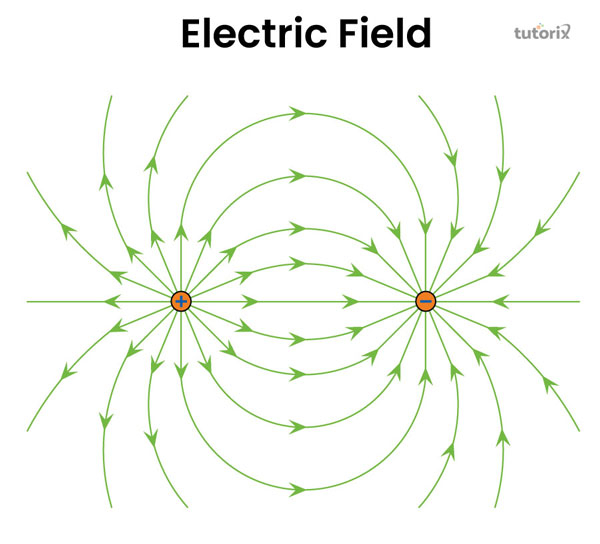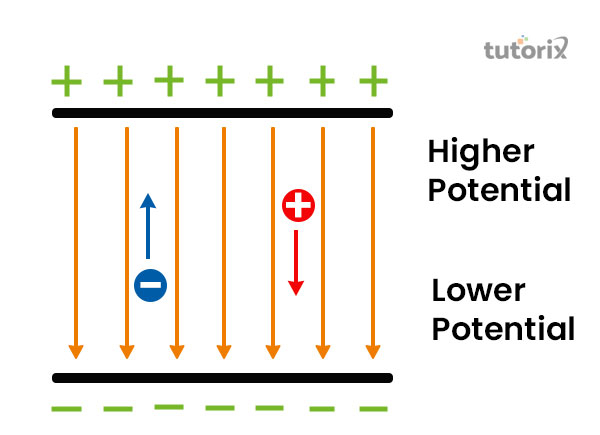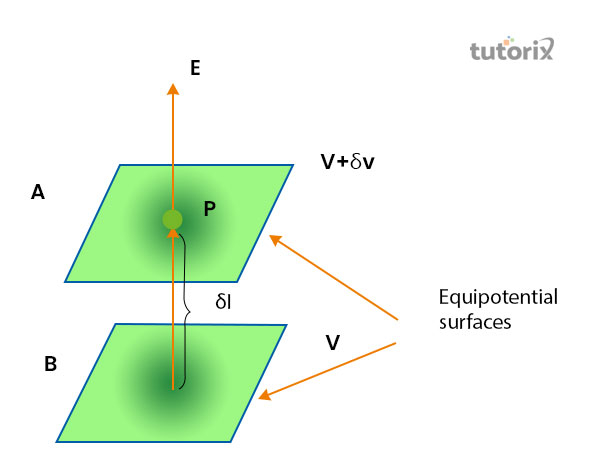# Relation between Electric Field and Electric Potential

## Introduction

The tutorial will be focused on an explanation of the definition of both the terms of physics, electric field followed by defining the term named electric potential. The tutorial will discuss how the information of charge at all points can be responsible for developing high electric potential where no electric field can exist. Based on this particular concept the relation between the electric field and electric potential will be included in this tutorial.

## What is An Electric Field?Figure 1: Electric field

Among the terminology of physics, electric field is conceptualised as a particular electric property that shares an associative relation with every point in the stretch of two electric charge points where the charge is present in any form (Benea-Chelmus et al. 2019).

Mathematically the definition of the electric field is as a vector field where on a positive test charge at every point of the space a positive force has been created which is refuted as the electric field. The direction and magnitude of the electric field are expressed by the value represented by the letter E, which refers to the intensity or strength of the electric field.

## What is Electric Potential?Figure 2: Electric potential

The total aggregation of work that is required for the displacement of a unit charge to a specific point from a point of reference against an electric field is referred to as the term electric potential. In order to say more accurately, the electric potential refers to the required energy for moving per unit charge in order to get a test charge that is as small as the disturbance of the space can be taken under consideration of negligibility (Fontanes et al. 2022). Based on this concept, the formula that is evolved is V = kq/r,

where the V represents the electric potential whereas k is the Coulomb constant. The distance of separation and the change are referred to by the letters respectively r and q.

## The Direction of the Electronic Field

The direction of the electric field is identified on the basis of two general concepts followed by the conceptualisation of electric potential and electric field. It has been stated that a positive direction can be taken if the direction of the field is identified as higher from a lower potential (Han et al. 2020). The second aspect that takes control over the direction specified on the electric field is that if the direction of the field is recorded to the lower potential from a higher one, the direction is taken as negative.

## Electric Field and Electric Potential: RelationFigure 3: Relation between electric field and potential

Generally, it is known that the electric field is considered a force that is produced by a charge that is placed near its surroundings. Inside this specific space, the amount of work is required in order to create a displacement of a unit positive charge to any specific point of the spec from the initial point is referred to as electric potential (Electricaltechnology, 2021).

This core relationship lies between these two different concepts of physics. In order to make this more understandable, it is needed to be taken into consideration that within the closely spaced two equipotential surfaces, A and B.

The values of potential are (V + δV) and V. as per the figure, the charge is δV and the direction of electric field is represented by V.

Based on these evaluations, the formula that is developed is |E| δl = V – (V + δV) = – δV.

## Identification of test charges based on electric gradient

In such cases where the electric gradients go higher towards the test charge, the identified test charge will be positive. On the other hand, if the scenario gets opposite, that is the movement of the electric gradient is lower and away from the test charge, the charge will be a negative one (Fontanes et al. 2022).

In contradiction in some cases, it can be seen that electric potential is placed at right angles to electric field lines, and the identified test charge will be found at the place that is the Equipotential surface.

## Conclusion

The present tutorial has shed light on a separate discussion of the definition of two concepts, electrical field and electrical potential. Based on this concept the tutorial has established a discussion to explain what kind of relation lies between these two aspects of physics.

It has been stated that two kinds of relational bond these two concepts shares, one of which states that the direction of the electric field will be towards there, where electronic potential decrease steepest. The second one state that the changes identified in the magnitude of electric potential are responsible for the change seen in the magnitude of electric field.

## FAQs

Q1. What is the electric potential difference?

The electric potential difference is generally known by the term voltage that can be defined by the required external work which is necessary to bring a change to another location from one in an electric field.

Q2. What is the difference between electric and magnetic fields?

The main difference that lies between these two terms is that the electric field is responsible to create an electric charge in the surroundings whereas, the magnetic field is responsible for creating an electric charge around the magnets that are moving.

Q3. What is the formula for the electric field?

The electric field is demonstrated as an electric property that is associated with every point in the expanse where the presence of charge is found in any form. In order to represent this concept as equivalent to the electric force per unit charge, the formula that is established is E = F /Q.

Q4. What is the relation between potential gradient and electric field?

The core relation shared between these two attributes states that the negative of potential gradient is equivalent to the intensity of electric field.

## References

### Journals

Benea-Chelmus, I. C., Settembrini, F. F., Scalari, G., & Faist, J. (2019). Electric field correlation measurements on the electromagnetic vacuum state. Nature, 568(7751), 202-206. Retrieved from: https://arxiv.org/pdf/1809.01785

Fontanes, P., Montanyà, J., Arcanjo, M., Urbani, M., Asensio, C., & Guerra-Garcia, C. (2022). On the Induced Currents to Wind Turbines by the Earth’s Atmospheric Electric Potential: Experiments With Drones. IEEE Access, 10, 21277-21290. Retrieved from: https://ieeexplore.ieee.org

Han, Y., Nickle, C., Zhang, Z., Astier, H. P., Duffin, T. J., Qi, D., ... & Nijhuis, C. A. (2020). Electric-field-driven dual-functional molecular switches in tunnel junctions. Nature materials, 19(8), 843-848. Retrieved from: https://par.nsf.gov/servlets/purl/10223636

### Website

Electrical4u.com, (2020). Electric Potential. Retrieved from: https://www.electrical4u.com/electric-potential/ [Retrieved on 11th June 2022]

Electricaltechnology.org, (2021). ELECTRICAL TECHNOLOGY. Retrieved from: https://www.electricaltechnology.org/2021/02/difference-between-electric-field-magnetic-field.html [Retrieved on 11th June 2022]

Updated on: 21-Aug-2023

623 Views